# 9.2.1 Scale Drawings, PT3 Focus Practice

Question 1:
Diagram below shows polygon P drawn on a grid of equal squares with sides of 1 unit.On the grid in the answer space, redraw polygon P using the scale
The grid has equal squares with sides of 1 unit.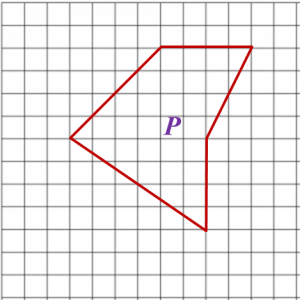Question 2:
Diagram below shows polygon W drawn on a grid of equal squares with sides of 1 unit.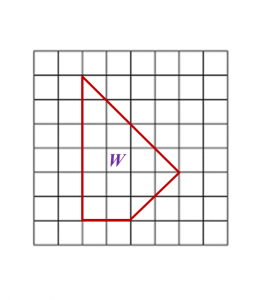On the grid in the answer space, redraw polygon W using the scale 1 : 2.
The grid has equal squares with sides of 1 unit.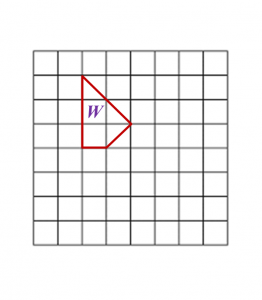Question 3:
Diagram below shows a polygon PQRSTU.(a) If the polygon is redrawn using the scale 1 : 400, calculate the length of PQ.
(b) In the answer space, redraw the polygon using the scale 1 : 400. The grid has equal squares with sides of 1 cm.

(a)

(b)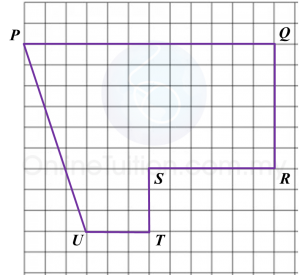Question 4:
Diagram below shows a polygon.On the grid in the answer space, redraw the polygon using the scale 1 : 500. The grid has equal squares with sides of 1 cm.

 Actual length Drawing 45 m = 4500 cm 4500 ÷ 500 = 9 cm 20 m = 2000 cm 2000 ÷ 500 = 4 cm 35 m = 3500 cm 3500 ÷ 500 = 7 cm 10 m = 1000 cm 1000 ÷ 500 = 2 cm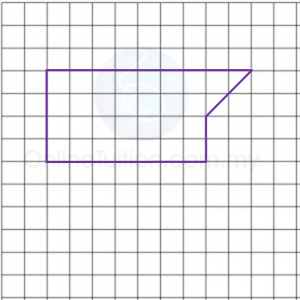Question 5:
Diagram below shows a polygon.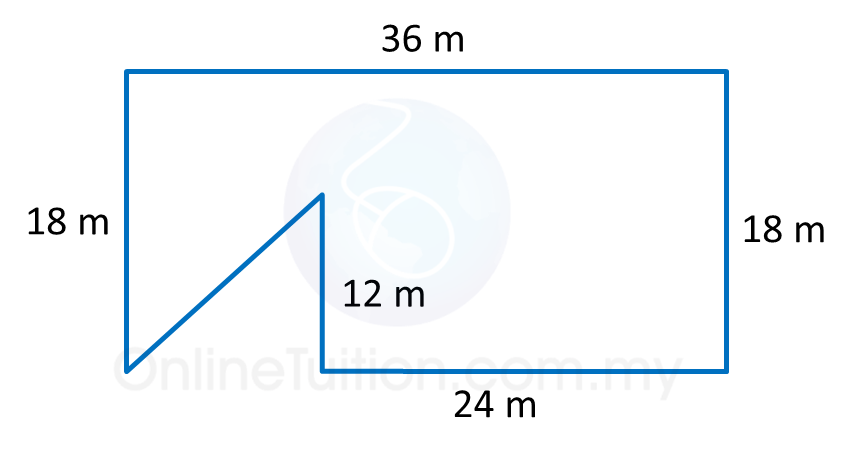(a) If the polygon is redrawn using the scale 1 : 300, calculate the length, in cm, the drawing for the side 24 m.
(b) In the answer space, redraw the polygon using the scale 1 : 300. The grid has equal squares with sides of 1 cm.